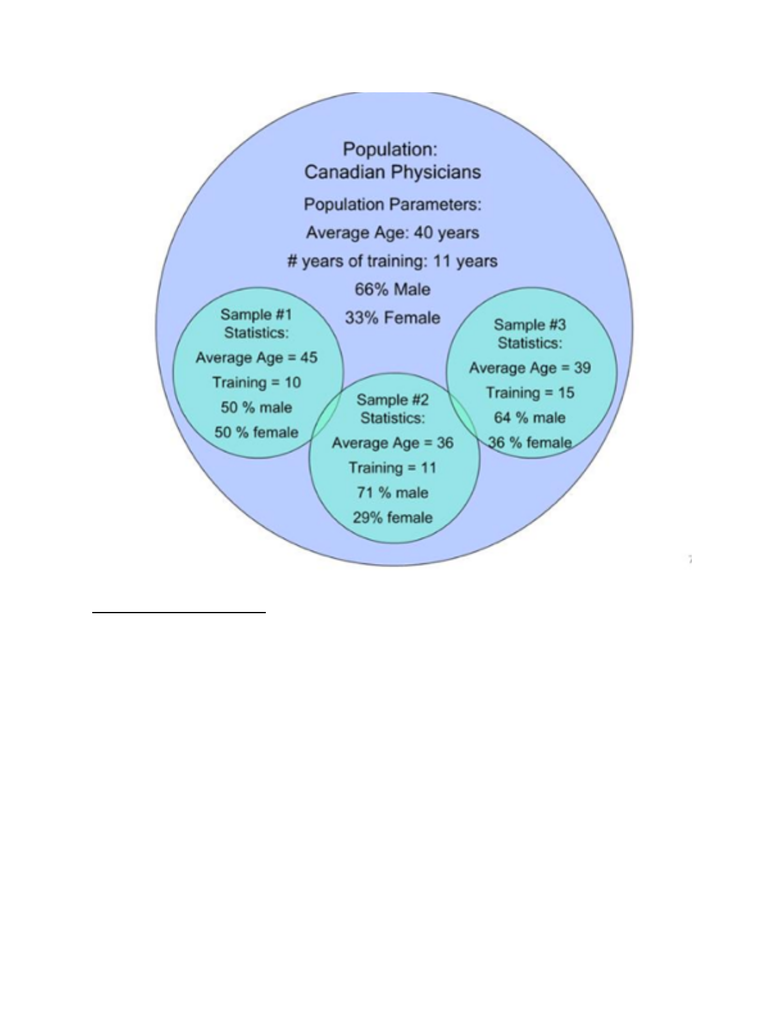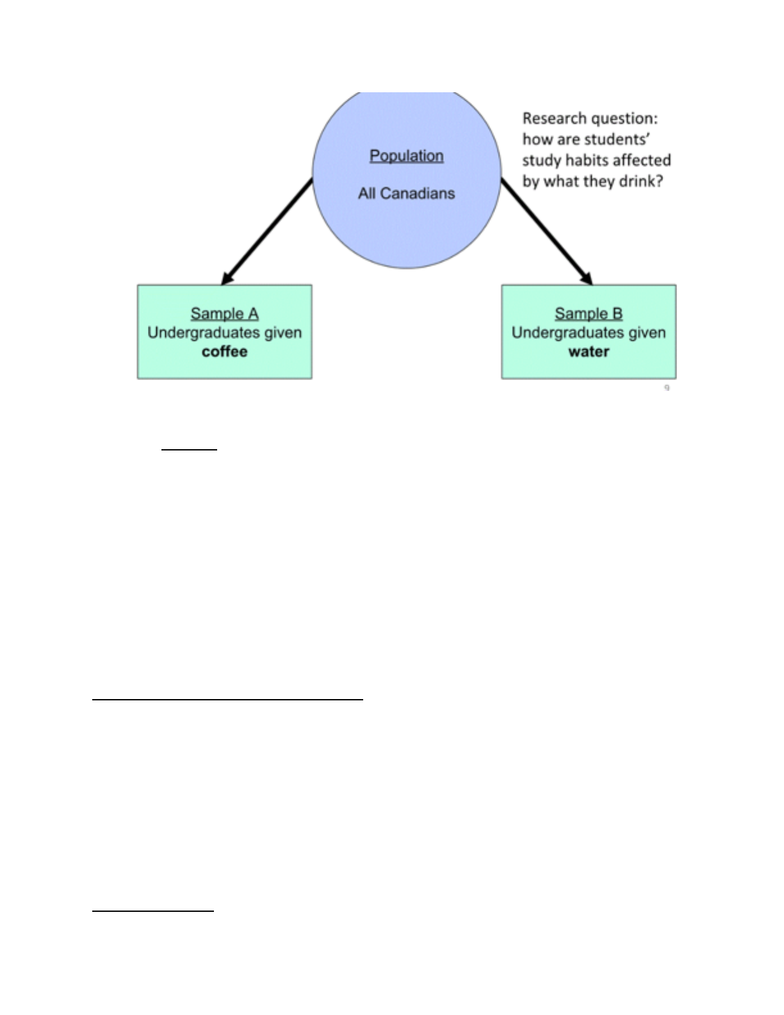Class Notes (1,100,000)
CA (620,000)
U of G (30,000)
PSYC (4,000)
PSYC 1010 (100)
Lecture 1

# PSYC 1010 Lecture Notes - Lecture 1: Statistical Inference, Statistic, Descriptive Statistics

Department
Psychology
Course Code
PSYC 1010
Professor
David Stanley
Lecture
1

This preview shows pages 1-3. to view the full 37 pages of the document.Statistics
The practice of collecting, analyzing, interpreting, and reporting
numerical data
Two Branches of Statistics
Descriptive statistics
o Organizes, summarizes, and communicates a group of
numerical information
Inferential statistics
o Using data collected from a representative group to draw
Descriptive Statistics
Tend to take the form of tables, graphs, or single numbers that
consolidate a large amount of information
Inferential Statistics
Techniques that use sample data to draw general conclusions about
the larger population
o Measuring everyone in the population is usually impossible
o Use sample statistics to infer what the population parameter
is likely to be
Populations and Samples
Population: the set of observation of interest in a particular study
Sample: A set of observations drawn from a population of interest
for inclusion in a study
Parameter: A (usually numerical) value that describes a whole
population
Statistic: A (usually numerical) value that describes a sample of the
population
Sampling Error
Sampling error is the _____ between a sample statistic and a
population parameter

Only pages 1-3 are available for preview. Some parts have been intentionally blurred.!
!
Basic Research Design
Pick a population of interest (e.g., students)
o Decide how you will recruit a sample (who, how many, etc…)
Pick a variable of interest (e.g., study habits)
o Decide how you will measure the variable
Turning Observations into Variables
o Observing people’s physical environment, attitudes, and
behaviour leads to variables – data that can take on different
values (vary)
Step 1: Define population and variables of interest

Only pages 1-3 are available for preview. Some parts have been intentionally blurred.!
!
Step 2: Descriptive Statistics
Results
Sample A: Average study time =
Sample B: Average study time =
!
Step 3: Inferential Statistics
Sample data show
Two possibilities
There is no real difference between the groups and sample
difference due to ________
There is a difference between the groups and the sample data
reflects this
Use _______ to decide between the two alternatives
!
Turning observations into Variables
Discrete variables
oVariables that can only take on only specific values (e.g.,
whole numbers)
oMeasures “how many”?
how many letters are in your name?”
Continuous variables
o Can take on a full range of values
o Measures “how much”?
“how tall are you?”
!
Always Discrete